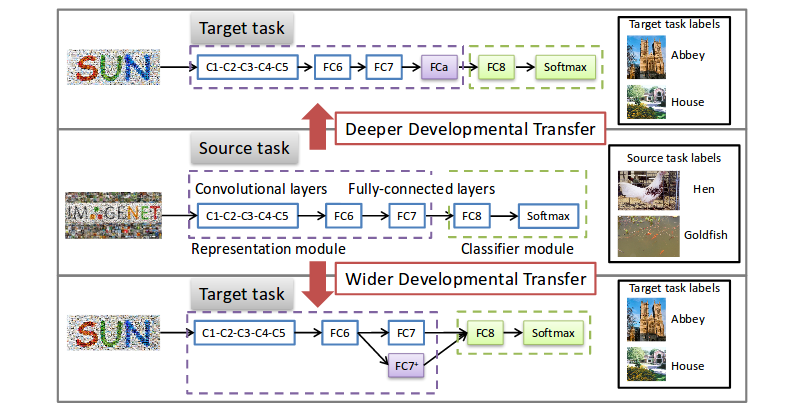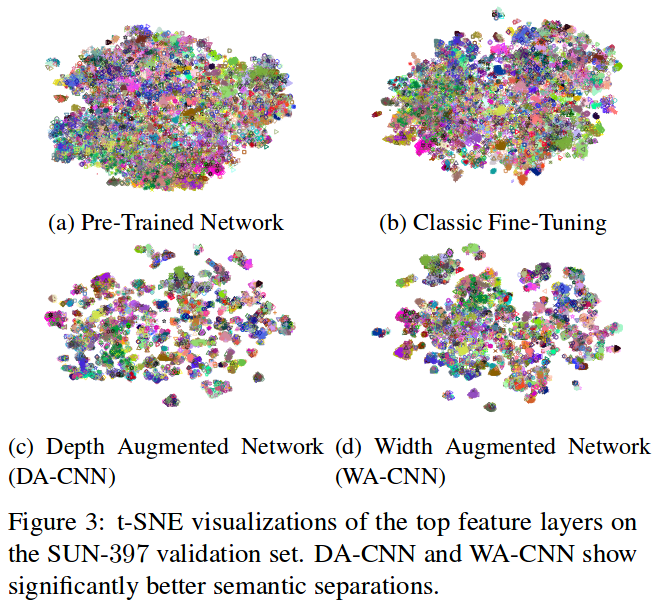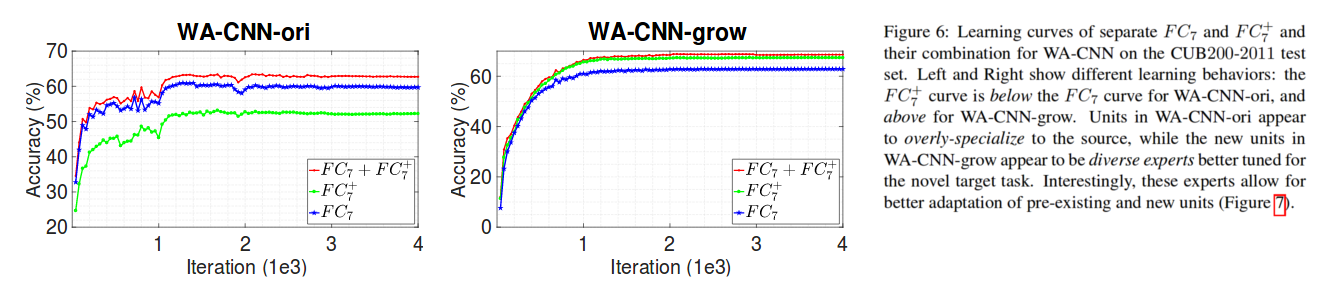In this paper, a developmental transfer learning methodology is introduced. The authors distinguish two types of developmental transfer, as you can see in the following figure. The violet boxes are newly-initialized conv layers, and the others have been pre-trained.# New neurons are slow learners

Because they just have been initialized, the new neurons have small parameters and activations. Thus, they will receive smaller error signals than their pretrained siblings during backprop, which is unfortunate. The authors state that this can result in a network that performs worse than the original pretrained one.

To reconcile the learning pace of the new and pre-existing units, the authors introduce an additional normalization and adaptive scaling scheme.

1. The activations of the old neurons and new neurons, $$h^k$$ and $$h^{k+}$$, are normalized like so:

$\hat{h}^k = h^k /||h^k||_2 \,, \enspace \hat{h}^{k+} = h^{k+} /||h^{k+}||_2$
2. The scales of the activations are now homogeneous, but they are also much smaller. As a remedy, they add an adaptive scaling operation:

$y^k_i = \gamma_i \hat{h}^k_i \,, \enspace y^{k+}_j = \gamma_j \hat{h}^{k+}_j$

where $$\gamma_i, \gamma_j$$ are learned by backprop. It is unclear how this operation interacts with batch norm, which itself introduces learnable feature-wise scaling.

# Experimental resultsAs you can see in the figure on the right, developmental transfer can help separate classes in the representational space.

The authors also state that their method help features capture more discriminative patterns. In the bottom figure, you can see the 5 maximally activating images for two selected features. The top row is a feature from the pretrained units, and the bottom row is an added feature. On the left, there is no normalization and scaling; on the right, the operations have been included.Next, the cooperative behavior of old and new units is studied.

Without the normalization and scaling (WA-CNN-ori), the new units are less useful for classification than the old. By adding the operations (WA-CNN-grow), we can see that the new units are more useful than the old!# Conclusion

• Increasing model capacity (and using normalization and scaling) significantly helps existing units better adapt and specialize to the target task.
• There is a slight but consistent benefit for widening the network, in comparison with deepening.
• State-of-the-art results have been obtained on the target datasets.